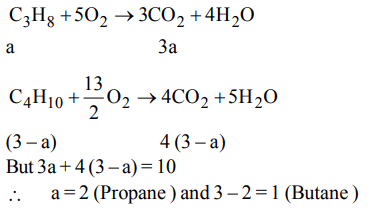## Some Basic Concepts of Chemistry Questions and Answers Part-10

1. If $3.01 ×10^{20}$  molecules are removed from 98 mg of $H_{2}SO_{4}$  , then the number of moles of $H_{2}SO_{4}$  left are
a) $0.1 ×10^{-3}$
b) $0.5 ×10^{-3}$
c) $1.66 ×10^{-3}$
d) $9.95 ×10^{-2}$

Explanation:2. 25.4 g of  $I_{2}$  and 14.2 g of  $Cl_{2}$  are made to react completely to yield a mixture of  $ICl$  and  $ICl_{3}$  . Calculate moles of  $ICl$  and  $ICl_{3}$  formed
a) 0.1, 0.1
b) 0.2, 0.2
c) 0.1, 0.2
d) 0.2, 0.1

Explanation:3. 2 g of a mixture of  $CO and CO_{2}$  on reaction with excess I2O5 produced $2.54 g of I_{2}$   . What be the mass % of $CO_{2}$ in the original mixture ?
a) 35
b) 70
c) 30
d) 90

Explanation: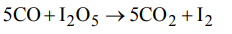4. The hydrated salt $Na_{2}CO_{3}.xH_{2}O$   undergoes 63% loss in mass on heating and becomes anhydrous. The value of x is
a) 10
b) 7
c) 5
d) 3

Explanation:5. Gastric juice contains 3.0 g of HCl per litre. If a person produces 2.5 litre of gastric juice per day. How many antacid tablets each containing 400 mg of $Al\left(OH\right)_{3}$  are needed to neutralize all the HCl produced in one day ?
a) 18
b) 14
c) 20
d) 17

Explanation: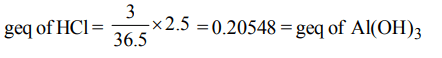6. Sulfuryl chloride $\left(SO_{2}CI_{2}\right)$  reacts with water to give a mixture of $H_{2}SO_{4}$ and HCl. How many moles of baryta would be required to neutralize the solution formed by adding 4 mol of $SO_{2}CI_{2}$  to excess of water ?
a) 1
b) 2
c) 3
d) 4

Explanation: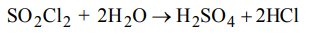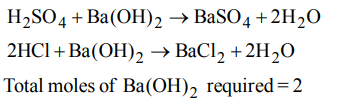7. A chloride of a metal (M) contain 65.5% of chlorine. 100 ml of vapour of the chloride of metal at STP weights 0.72 g. The molecular formula of the metal chloride is
a) $MCl_{4}$
b) $MCl_{3}$
c) $MCl_{2}$
d) MCl

Explanation: Molecular weight of metal chloride8. 7.36 g of a mixture of KCl and KI was dissolved in $H_{2}O$  to prepare 1 litre solution. 25 ml of this required 8.45 ml of 0.2 N $AgNO_{3}$  , what are % of KI in mixture ?
a) 57.28
b) 47.28
c) 5.72
d) 49.12

Explanation: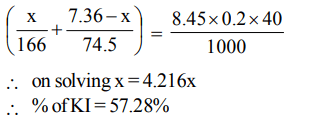9. When burnt in air, 14.0 g mixture of carbon and sulphurgives a mixture of  $CO_{2} and SO_{2}$  in the volume ratio of 2 : 1, volume being measured at the same conditions of temperature and pressure moles of carbon in the mixture is
a) 0.75
b) 0.5
c) 0.40
d) 0.2510. A gas mixture of 3 litres of propane $\left(C_{3}H_{8}\right)$  and butane $\left(C_{4}H_{10}\right)$  on complete combustion at 25° C produced 10 litre $CO_{2}$ . Find out the composition of gas mixture (Propane : Butane)